PSA2RWT - Classwork---Geometry, M1, Lesson 34 (G.CO.A.1, G.CO.C.9, G.CO.C.10, G.CO.C.11))

1. Assumption/Fact/Property: Given two triangles ABC and A'B'C' so that AB = A'B' (Side), m∠A = m∠A' (Angle), and AC = A'C' (Side), then the triangles are congruent. [SAS]

The figure below is a parallelogram ABCD. What parts of the parallelogram satisfy the SAS triangle congruence criteria for ΔABD and ΔCDB? Describe a rigid motion(s) that maps one onto the other. (Consider drawing an auxiliary line.)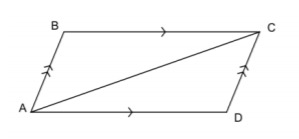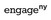Modified from EngageNY ©Great Minds Disclaimer

2. Assumption/Fact/Property: Given two triangles ABC and A'B'C', if m∠A = m∠A' (Angle), AB = A'B' (Side), and m∠B = m∠B' (Angle), then the triangles are congruent. [ASA]

In the figure below, ΔCDE is the image of the reflection of ΔABE across line FG. Which parts of the triangle can be used to satisfy the ASA congruence criteria?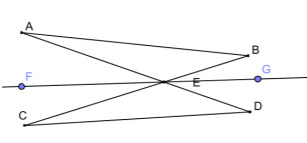Modified from EngageNY ©Great Minds Disclaimer

3. Assumption/Fact/Property: Given two triangles ABC and A'B'C', if AB = A'B' (Side), AC = A'C' (Side), and BC = B'C' (Side), then the triangles are congruent. [SSS]

ΔABC and ΔADC are formed from the intersections and center points of circles A and C. Prove ΔABC ≅ ΔADC by SSS.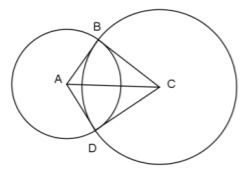Modified from EngageNY ©Great Minds Disclaimer

4. Assumption/Fact/Property: Given two triangles ABC and A'B'C', if AB = A'B' (Side), m∠B = m∠B' (Angle), and m∠C = m∠C' (Angle), then the triangles are congruent. [AAS]

The AAS congruence criterion is essentially the same as the ASA criterion for proving triangles congruent. Why is this true?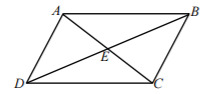Modified from EngageNY ©Great Minds Disclaimer

5. Assumption/Fact/Property: Given two right triangles ABC and A'B'C' with right angles ∠B and ∠B', if AB = A'B' (Leg) and AC = A'C' (Hypotenuse), then the triangles are congruent. [HL]

In the figure below, CD is the perpendicular bisector of AB, and ΔABC is isosceles. Name the two congruent triangles appropriately, and describe the necessary steps for proving them congruent using HL.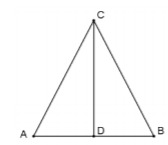Modified from EngageNY ©Great Minds Disclaimer

6. Assumption/Fact/Property: The opposite sides of a parallelogram are congruent.

The opposite angles of a parallelogram are congruent.

The diagonals of a parallelogram bisect each other.

In the figure below, BE ≅ DE and ∠CBE ≅ ∠ADE. Prove ABCD is a parallelogram.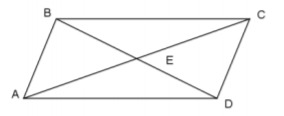Modified from EngageNY ©Great Minds Disclaimer

7. Assumption/Fact/Property: The midsegment of a triangle is a line segment that connects the midpoints of two sides of a triangle; the midsegment is parallel to the third side of the triangle and is half the length of the third side.is the midsegment of ΔABC. Find the perimeter of ΔABC, given the labeled segment lengths.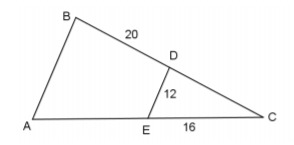Modified from EngageNY ©Great Minds Disclaimer

Type your answer below as a number (example: 5, 3.1, 4 1/2, or 3/2):
Part A)

8. Assumption/Fact/Property: The three medians of a triangle are concurrent at the centroid; the centroid divides each median into two parts, from vertex to centroid and centroid to midpoint, in a ratio of 2: 1.

a) If,, andare medians of ΔABC, find the length of BG given the labeled lengths.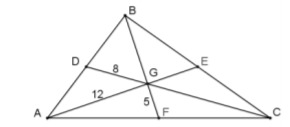Modified from EngageNY ©Great Minds Disclaimer

Type your answer below as a number (example: 5, 3.1, 4 1/2, or 3/2):
Part B)

b) Based on the figure in Part (a), find the length of GE.

Type your answer below as a number (example: 5, 3.1, 4 1/2, or 3/2):
Part C)

c) Based on the figure in Part (a), find the length of CG.

Type your answer below as a number (example: 5, 3.1, 4 1/2, or 3/2):